# Introduction to Calculated Columns in SharePoint

In the previous article, I have explained about Hyperlink and Picture column type. We have seen how to use this column and also, I have compared the Single line of text column type with Hyperlink and Picture Column types. In this article, I am going to explain Calculated column types available in the SharePoint list.

In this article, I am going to cover the following topics in detail.

• Background
• Introduction to Calculated Columns
• Creating and configuring Calculated Columns
• Summary

Background

Consider a scenario in which you are working with Excel and you are using the formula, such as =E3 + F3. So what will this do? This will add the values of cells E3 and F3 and display the sum in the column in which you have applied this formula. This was the basic requirement, now consider another example where you have the price of the item in the column and you are entering the quantity purchased and the total price has been auto-populated in an Excel column (=Qty Column address * price column address). So this is called calculated formulas which depend on the other cell values or certain predefined values.

Now come back to SharePoint, you have a list in SharePoint which manages your shopping total. And you have the price of the item columns, quantity columns, and Total price columns. Now what you will do, you will first enter the price of the product, then quantity and then you will multiply both and then enter the total price into the Total price column. This is not the wrong approach; this is also a correct approach. But the problem is, here you have to manage your calculations manually, and where there is manual interference, the data may contain errors as we humans make errors with calculations. So what to do? So in this article, I will be solving this problem using calculated column types available in SharePoint. SharePoint also provide the replication of Excel formulas in terms of Calculated Columns. What are Calculated Columns and how are they used? Let’s explore that in detail.

Introduction to Calculated Columns

Calculated column is defined as “This special column type allows a site manager to define a formula that will automatically determine the value of a column without requiring a user’s input. This formula can be based upon other columns within the list. An example of such a formula is [Items Sold] × [Price], which would calculate the total revenue associated with a list item that represents a customer sale” (The definition is taken from the book “Beginning SharePoint 2013.pdf”). As per the definition, it is clear that we can combine two or more than two columns to produce the output in the calculated column.

Creating and configuring Calculated Columns

Before jumping into the creation of columns, let’s imagine a scenario in which we have a SharePoint custom list with name “Shopping”. The list contains the columns such as Price, Quantity, Total Price. Here Total Price will be containing a value from Price * Quantity. Here is the list structure.Now, let’s create a new column “Total Price”. This column will be a calculated column. You can create a column by selecting “Calculated” in the column type. Now, you will have the following option on your screen.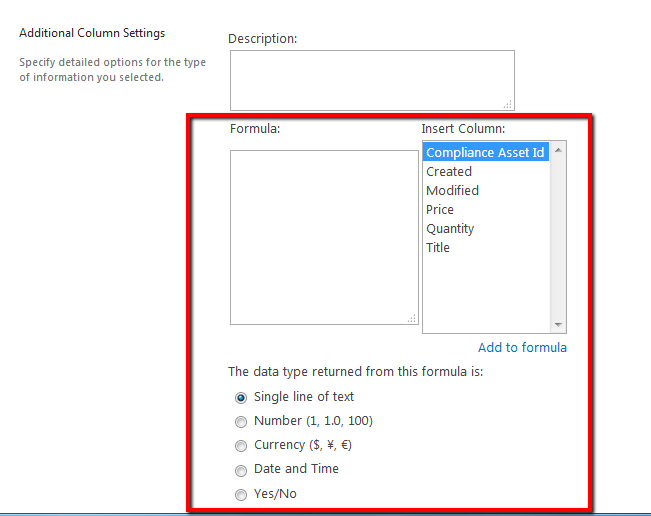Formula: Here you have to build the formula. The formula in our case will be like (=Price * Quantity). So, please follow the below steps to configure the formula.

• Step-1: Type “=” in the Formula section.
• Step-2: Double click on “Price” column from “Insert Column”
• Step-3: Type “*” for multiplication
• Step-4: Double click on “Quantity” column from “insert Column”

Your final formula will look like this.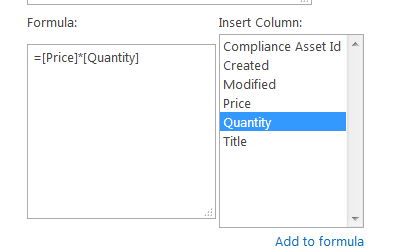The data type returned from this formula is  the following column types.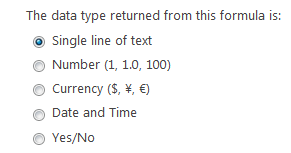The column types which are shown here will be the output of the calculation. Here, I have selected Single line of text; then the calculated column will be created in Single line of text type.

Once you’ve got every configuration, click on OK. This will create a new column, “Total Price”, in the list.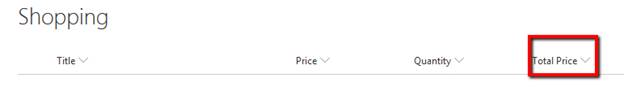Now, let’s add the item in the list. Here, I am entering 50 in Price column and 10 in Quantity.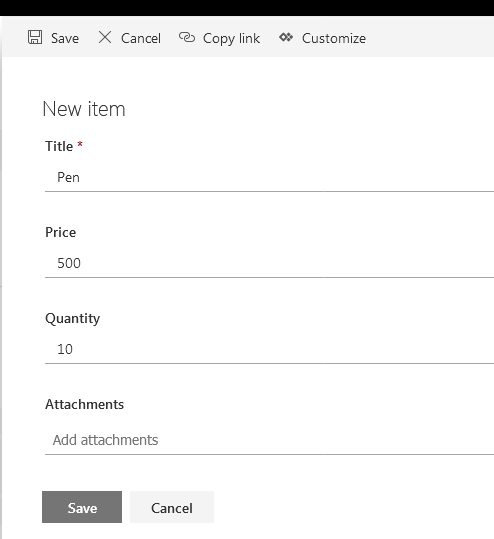You will note here that the column “Total Price” is not visible in the form. That’s the power of the calculated column. You cannot edit the value for calculated columns directly in SharePoint. If you want to edit the value of the columns, then in that case you have to edit the values in the referenced columns. Once you have entered the value, click on Save. Once you will save the form then you will find the following values in the list. In Total Price, we will have 5000 (500*10).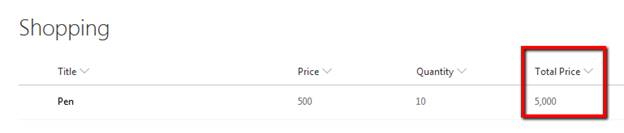This was the basic formula. In calculated columns, we can insert advanced level formulas too. There are several articles written on this column types with have multiple formula configured. Here I am sharing a few of the articles.